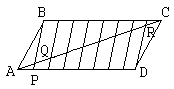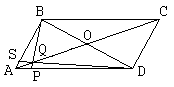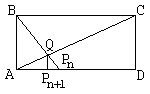# How to divide a segment into n equal parts

The realm of mathematics is vast, even if we only talk of elementary mathematics. So I received it as a compliment when Monty Phister, the author of a popular CD-ROM Gnarly Math, complained to me recently of the absence at my site of a wonderful construction discovered by two young students Dan Litchfield and Dave Goldenheim in 1995. I interpreted Monty's inquiry as a wonderment how such a comprehensive resource as this site managed to eschew a Description of an interesting topic and that of a recent event of some educational significance.

The reason was partly in that, since the publication of the report on the discovery in Mathematics Teacher in January 1997, it became impossible to discuss the topic without relating to the shallow publicity that accompanied the wonderful discovery. The advent of new technology and new software has a profound effect on the whole of instructional process. We may expect in the future that much of mathematics usually expounded by the teacher in classroom will be independently discovered by students assisted by the new technology. Such private discoveries and personal involvement, it is hoped, will enhance students' motivation in a particular subject or, more generally, in the knowledge acquisition as a whole.

The same facts will be discovered all over again by millions of students. Which should not diminish in the least each one's sense of private accomplishment. This is exactly the expected and the widely desired outcome of the education reform that students should learn mathematics via doing it. It's quite probable that, now and then, students will make novel discoveries.

Without detracting from Dan and Dave's personal achievement, it must be said that this was not what happened in their case. The boys deserve all the praise for discovering a non-trivial result. It's embarrassing, nonetheless, for the teaching community which apparently did not recognize a well known construction even after a dozen invited talks at various NCTM conferences.

During a Summer Geometry course at Greens Farms Academy, Connecticut, the two boys were asked to devise a procedure for dividing a given line segment into n equal parts. The teacher, Charlie Dietrich, anticipated a variation on the standard construction based on the venerable Euclid's Proposition VI.9. To his surprise, "Within two hours, they announced that they had solved it, not with compass and straightedge but on a computer by using The Geometer's Sketchpad."

Somehow, the construction was perceived as a novel achievement, one of a kind in 2500 years from publication of the Elements. The news got around. Invitations to conferences followed. I may be mistaken, but not until January 1997, when the report has been published in Mathematics Teacher and noticed by Jim Wilson from the University of Georgia, the discovery has been set in a historical context.

Personally, I was aware of the construction as a school boy. The basic lemma below appears as Problem #1.4 in a problem collection by Prasolov (Moscow, 1986). In [Golovina and Yaglom], p. 50 it appears as Example 23 along with the remark that the whole construction can be accomplished with a straightedge alone. Following is my recollection from many years ago. Jim Wilson offers a list of sites that tackle the problem of division of a segment into n parts.The following lemma serves as the basis for an inductive construction of the nth part of a given segment.## Lemma

Let the point P on the side AD of a parallelogram ABCD be such that n·AP = AD. Let Q be the point of intersection of AC and BP. Then (n + 1)·AQ = AC.

### Proof 1

From the similarity of ΔAPQ and ΔBQC, QC/AQ = BC/AP = AD/AP = n. Therefore, AC/AQ = n + 1.### Proof 2

Divide AD and BC into n equal parts. Through the division points (of which P is one) draw lines parallel to BP. Let R be the intersection point on the diagonal AC opposite Q. By construction (or by Euclid VI.9), the side QC of ΔBQC is divided into n equal parts. The same is true of the side AR of ΔADR. Thus it is obvious that the diagonal AC is divided into n + 1 equal parts.### Proof 3

Draw the diagonal BD. Let O be the point of intersection of the two diagonals. Draw also the line DS through Q. We shall apply Ceva's theorem to ΔABD. Since BO = OD and AS/SB · BO/OD · DP/AP = 1, we have AS/SB = AP/DP = 1/(n - 1). By the VanObel theorem, AQ/QO = AS/SB + AP/DP = 2/(n - 1), or AQ/AO = 2/(n + 1). Since AC = 2AO, AQ/AC = 1/(n + 1).

## Remark 1

Proof 3 shows how Lemma can be easily generalized. If AP/AD = a/b, for some real a and b, then AQ/AC = a/(a + b).

From now on, to simplify the diagrams, I'll be referring to rectangles, although all results (after obvious modifications) remain valid for general parallelograms.## Corollary 1

Apply Lemma to a rectangle ABCD. Let APn be the nth part of the side AD. Find Q at the intersection of BPn and AC. Let Pn+1 be the foot of the perpendicular from Q to AD. Then (n + 1) · APn+1 = AD.

### Proof

The assertion follows from Lemma and the similarity of triangles ACD and AQPn+1.## Theorem

Assume in a rectangle ABCD, BM/BC = a/b and AP/AD = c/d. Let R be the intersection of AM and BP, and S the foot of perpendicular from R to AD. Then AS/AD = ac/(ad + bc).

### Proof

To obtain this result, first apply Remark 1 to the diagonal BD (instead of AC) and the point M (instead of P). Then use Ceva's theorem and the VanObel theorem in ΔABD as in Proof 3 of Lemma. This yields BR/BP. Finally, consider similar triangles ABP and SPR with the view to Euclid VI.9.

## Corollary 2

Let in Theorem a = c = 1. Then AS/AD = 1/(b + d).

This leads to the following construction. Start with b = 1 and d = 2. The theorem yields the ratio 1/3. Move P to BC to replace M, and use S instead of P on AD. This leads to b = 2 and d = 3. So the theorem yields the ratio 1/5. Repeat this process now with b = 3 and d = 5. The next ratio will be 1/8, and so on. In which manner we can generate the whole of the Fibonacci sequence.## Generalization

The lemma at the top of the page is a particular case of the following problem that has been published (Geometry problem #90) in 1965. Let a straight line l intersect sides AB and CD of a parallelogram ABCD at points E and F, respectively. Let G be the intersection of l with the diagonal AC. Then AB/AE + AD/AF = AC/AG. The lemma is obtained when E coincides with B.

## Remark 2

It is obvious that the GLaD construction can be carried out by paper folding. As such, it is known as the crossing diagonal method. It was described by R. J. Lang in 1988.

### References

1. L. Y. Golovina and Y. M. Yaglom, Induction in Geometry, Fizmatgiz, 1961, in Russian
2. R. J. Lang, Four Problems III, British Origami, no 132, October 1988, pp. 7-11
3. A. A. Leman, Problems from the Moscow Mathematical Olympiads, Prosveshchenie, 1965, in Russian
4. V. V. Prasolov, Problems in Planimetry, v 1, Nauka, Moscow, 1986 , in Russian